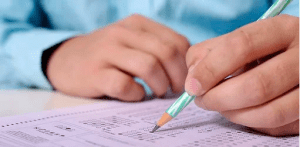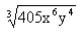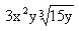# Hardest ACT Math Questions

When it comes to preparing for your future, few things open doors more quickly than high standardized test scores. A great ACT score can mean the difference between getting accepted to your dream school or having to go to college elsewhere, receiving scholarships or taking out student loans, and so much more. As such, it is crucial that you do everything possible to try to increase your ACT score as much as possible before you head off for college.

The biggest obstacle standing in the way of a great score for many test-takers is the ACT Math section. With 60 questions to tackle in a limited time, many high schoolers worry about how they can master the math section and get their desired ACT score.

If you are one of these students, you do not need to fret! Unlike some other sections of the ACT, the ACT Math section only covers a limited number of topics, making it one of the easiest sections to prepare for, even if math is not your forte.Once you are aware of the types of questions you will face on the ACT Math section, you will be able to practice and eventually get the score you need.

Here are the top 10 hardest ACT Math practice questions you should master if you want to ace the math section of the ACT:

## 1. Data representation

The ACT Math section requires you to be able to read and interpret charts, graphs, tables, and other figures. You may have to calculate a ratio based on information provided in a pie chart or choose a graph that adequately represents the decay of radioactive substance, for instance.
When you prepare for the ACT Math section, make sure you have plenty of data representation practice problems.

## 2. Linear equations

Make sure your ACT Math practice also includes questions that ask you to solve linear equations.

You might see questions like: If 8 – 3x = 14, then 2x = ?

In order to get to the correct answer, -4, you will need to know how to solve single variable linear equations.

While these types of questions might not seem too difficult, there are very common on the math section of the ACT, and they can cause you a lot of trouble if you do not add these questions to your daily practice.

## 3. Algebraic operations and inequalities

Some questions in the ACT Math section will ask you to move beyond solving linear equations and solve problems using several variables and higher-order terms.

Expect to see questions that ask you to combine like terms, tackle algebraic inequalities, and multiply binomials.

A challenging practice question that these skills might look like this:

Which of the polynomials below is equivalent to (6x – 3) (2x + 1)?

A. 12×2 – 12x – 3
B. 12×2 + 12x – 3
C. 12×2 + 6x – 3
D. 12×2 – 6x – 3
E. 12×2 – 3

In order to find the correct answer, which is option E, you will need to use the FOIL method.

## 4. Logarithms

Logarithms are a form of intermediate algebra that you will see on the math section of the ACT. Before you take the test, you should be familiar with the properties of logarithms and know how to re-write any exponential equation into logarithmic form (and re-write any logarithm into an exponential equation, as well).

For example, solve 2(log x) – log(7x-1) = 0.

This will require you to combine the logarithms, re-write them in exponential form, use the quadratic formula, and plug these numbers into the original equation to end up with x= 0.1459 and x=6.8541.

Practice problems like the one above will help you prepare for these questions on the ACT.

## 5. Sequences and patterns

While preparing for the ACT Math section, make sure you practice a question or two that involves sequences and patterns. These types of questions will typically involve you knowing arithmetic sequences and geometric sequences.

For example, The sum of five consecutive integers is 505. What is the third number in this sequence?

The answer to this practice problem is 101 just in case you were wondering!

## 6. Slope

You probably already expect that you will need to know the equation for slope (y=mx+b) when it is time for you to take the test; however, you will need to be able to do a lot more than understand the formula to get a high score.

Make sure you practice finding the slope between two points on a graph, rearranging equations to find the slope, and calculating the slope of any given line.

## 7. Graphing trigonometric functions

The ACT Math section may have a healthy dose of trigonometry. You should be prepared to graph trigonometric functions, use radians instead of degrees to make calculations, understand sin, cos, and tan functions, and know SOHCAHTOA.

You should also practice shrinking and stretching these trigonometric functions.

## 8. Plane geometry

There are a few topics within plane geometry that you will want to master before test day. At the very least, you should practice questions involving volume and 3D geometry, using the Pythagorean theorem to calculate the angles of different triangles, identifying transformations of shapes within a plane, and understanding the properties of different polygons.

For example, In hexagon ABCDEF, one interior angle measures 40°. What is the total measure of the remaining 5 interior angles?

A. 200°
B. 400°
C. 500°
D. 660°
E. 680°

You will need to know the equation for finding the sum of interior angles in order to determine that 680° is the correct answer.

## 9. Polynomials

When you reach the part of the ACT Math section that focuses on coordinate geometry, you will be asked to solve a variety of problems, especially ones featuring polynomials.

When you take ACT Math practice tests, make sure you have questions that ask you to identify graphs of different polynomials, modify polynomial equations, and identify whether a given polynomial function is odd, even, or neither.

For instance, Is the following function odd, even, or neither: f(x)=−4x^5+7x^3?

With enough practice, you will be able to determine that f is odd in this instance, and you will be able to solve similar problems on the ACT.

For example, a strong practice question may ask you to simplify a radical like thisso that you end up with this:.